Math Worksheets for Kids Home > Pre-made Math Worksheets - Subtraction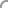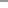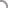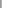Welcome Guest User
 • Spotlight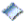New Worksheets...Easter Worksheets...Custom Search
 Pre-made Worksheets: By Grade  — Pre-K and Kindergarten  — 1st Grade  — 2nd Grade  — 3rd Grade  — 4th Grade  — 5th Grade   By ThemeValentine's DaySt. Patrick's DayEasterSpringSummerFallHalloweenThanksgivingChristmasNew Year   By OperationCounting/ Skip CountingComparisonAdditionSubtractionMultiplicationDivisionMatching   By Special Topics/ Skills  — Basic Numbers  — Number Lines  — Fact Family  — Place Value   — Time   — Thermometer   — Money  — Even/Odd Numbers   — Roman Numbers  — Prime Numbers  — Sort/Order Numbers  — Measurement  — Color By Number  — Word problems  — Ordinal Number & Names  — Rounding  — Percentage  — Fractions  — Decimals   — Algebra  — Shapes/Geometry  — Math Drills Custom Worksheets: • Standard Operators    Addition/Subtraction    Multiplication/Division • Number Tables • Math Fact Table Interactive Flash Cards • Multiplication • Addition Other Activities • Quiz/Assessments Contact Us • Suggestions Notify Me >> Enter Email: Testimonial ... Fabulous! My son didn't understand Fact Families at school - and after I found your site, he mastered the concept very quickly... Texas, USA Tell us what do you think about our site.>>
 Explore Schools in USA AboutUs.org Page MathCentral4Kids Site Federal Education Regulations California Law on Education Other sites: A B C D FR F G H IAirlines Scottsdale Deals Privacy Policy Terms of Use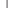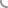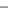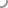Subtraction Worksheets

Select a Worksheet

Categories:
 1. Number Line - Subtraction 2. Subtract using  pictures 3. Subtract using pictures or numbers 4. Subtract and Color by Number 5. Subtract a number 6. Subtract Temperatures (US Cities) 7. Number Sentence - Subtraction 8. Subtraction - Word Problems 9. Fact Equivalents 10. Subtraction - Decimal Numbers 11. Mixed - Add or Subtract 12. Mixed - Add and Subtract - Solve the sequence 13. Puzzle - Solve by Addition and Subtraction 14. Add or Subtract and Color by Number 15. Add, Subtract or Multiply and Color by Number 16. Fact Family - Addition and Subtraction 17. Drills - Mixed Operators - Addition, Subtraction 18. Mixed - Imaginative Problems 19. Drills - Mixed Operators - Addition, Subtraction, Multiplication 20. Drills - Mixed Operators - Addition, Subtraction, Multiplication, Division Note: - Click here for Spring Math Worksheets.- Click here to create Custom and Mixed Worksheets. - Click here for Math Drills Worksheets.

 1 Number Line - Subtraction Top

Simple Line
Range 0 to 10
Range 0 to 20
Range -10 to 10

 2 Subtract using  pictures Top

(No Borrowing/Trading)
Single Digit
Worksheet #1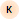(Theme: Valentine - Heart) [4 questions]
1-2 Digits
Worksheet #1(Theme: Christmas - Tree) [4 questions]
Worksheet #2(Theme: Christmas - Ornament) [4 questions]
Worksheet #3(Theme: St. Patrick's  - Shamrocks) [5 questions]

 3 Subtract using pictures or numbers Top

(No Borrowing/Trading)
Single Digit

 4 Subtract and Color by Number Top

Standard
Single Digit
Worksheet #1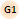(Theme: Thanksgiving - Turkey) [9 questions]

Based on Even/ Odd
Single Digit

 5 Subtract a number Top

Subtract One
Worksheet #1(Theme: Halloween - Pumpkin) [9 questions]
Worksheet #1(Theme: Christmas - Ornaments) [9 questions]

Subtract Two
Worksheet #1(Theme: Christmas - Ornaments) [8 questions]

(No Borrowing/Trading)
Single Digit - With theme picture
Worksheet #1(Theme: Halloween - Turkey) [8 questions]
Worksheet #2(Theme: Halloween - Witches Hat) [8 questions]
Worksheet #3(Theme: Halloween - Ghost) [8 questions]
Worksheet #4(Theme: Halloween - Candy Corn) [8 questions]
Worksheet #5(Theme: Christmas - Tree) [10 questions]
Worksheet #6(Theme: Christmas - Snowman) [10 questions]
Worksheet #7(Theme: Christmas - Tree) [10 questions]
Worksheet #8(Theme: Christmas - Snowman) [10 questions]
Worksheet #9(Theme: Valentine - Heart) [10 questions]
Worksheet #10(Theme: St. Patrick's  - Shamrocks) [10 questions]
Single Digit
Two Digit
Three Digits

(With Borrowing/Trading)
Two Digit
Worksheet Dynamic #4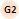(Theme: Christmas - Ornaments) [20 questions]
Three Digits

 6 Subtract Temperatures (US Cities) Top

(No Borrowing/Trading)
Two Digit

(Some with Borrowing/Trading)
2 to 3 Digits

 7 Number Sentence - Subtraction Top

Single Digit
Worksheet #1(Theme: Thanksgiving - Turkey) [3 questions]

 8 Subtraction - Word Problems Top

Single Digit
a + ? = c => c - a = ?
Mixed

Two Digits

Additional Word Problems Worksheets

 9 Fact Equivalents Top

Addition & Subtraction

 10 Subtraction - Decimal Numbers Top

Tenths (Level A)

Hundredths (Level A)

Tenths (Level B)

Hundredths (Level B)

Thousandths (Level B)

Mixed (T, H, Th) (Level C)

 11 Mixed - Add or Subtract Top

Single Digit

 12 Mixed - Add and Subtract - Solve the sequence Top

 13 Puzzle - Solve by Addition and Subtraction Top

 14 Add or Subtract and Color by Number Top

Single Digit
Worksheet #1(Theme: Christmas - Bulbs) [18 questions]

 15 Add, Subtract or Multiply and Color by Number Top

Single Digit

 16 Fact Family - Addition and Subtraction Top

Level A
Set 1
Set 2

Level B
Set 1
Set 2
Set 3

Level C

Additional Fact Family Worksheets

 17 Drills - Mixed Operators - Addition, Subtraction Top

Single Digit

 18 Mixed - Imaginative Problems Top

Addition/Subtraction
Tree Arrangement
Worksheet #1(Theme: Thanksgiving - Turkey) [10 questions]

 19 Drills - Mixed Operators - Addition, Subtraction, Multiplication Top

Single Digit

 20 Drills - Mixed Operators - Addition, Subtraction, Multiplication, Division Top

Single Digit

 Want to get notified about new myWeblets worksheets?
Search for Worksheets: